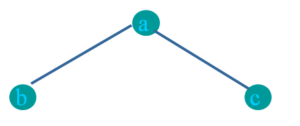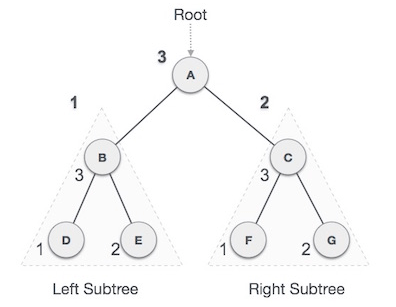# Traversal

Traversal is a process to visit all the nodes of a tree and may print their values too. Because, all nodes are connected via edges (links) we always start from the root (head) node. That is, we cannot randomly access a node in a tree. There are three ways which we use to traverse a tree −

• In-order Traversal
• Pre-order Traversal
• Post-order Traversal

Generally, we traverse a tree to search or locate a given item or key in the tree or to print all the values it contains.

## Pre-order Traversal

In pre-order first visit Root node then visit left subtree and finally right subtree.

Root-Left-Right

#### Algorithm

```Step 1: Visit root node.
Step 2: Traverse left subtree(Recursively).
Step 3: Traverse right subtree (Recursively).```
```Pre-order: abc
Example:```

We first visit A the root node and then move to its left subtree B,  B is also a root node of D&E so first print B then its left D and right E.  The same is repeated for right sub tree C , C is root of F&G The process goes on until all the nodes are visited.

Pre-order: A → B → D → E → C → F → G

Example

Pre-order: abdgheicfj
Example

Pre-order:/*+ab-cd+ef

## Pre-order traversal code

Void Preorder ( TreeNode <int> *treeNode )

{

if ( treeNode != Null)

{

Cout<< *(treeNode -> getInfo () ) << “  “;

Preorder ( treeNode -> getleft() );

Preorder (treeNode -> getRight() );

}

}

## In-order Traversal

In this traversal method, the left subtree is visited first, then the root and later the right sub-tree.

Left-Root-Right

In-order: bac

#### Algorithm

```Step 1: Traverse left subtree (Recursively).
Step 2: Visit root node.
Step 3: Traverse right subtree(Recursively).```

We start from A, A is root so travers to left sub tree B, B is also a root of D&E again traverse to left. First print D then its root B and right E.  DBand E is all left of Root A so after these print A and traverse right sub tree as same.

D → B → E → A → F → C → G

Example:

## In-order traversal code

Void Inorder ( TreeNode <int> *treeNode )

{

if ( treeNode != Null)

{

Inorder ( treeNode -> getleft() );

Cout<< *(treeNode -> getInfo () ) << “  “;

Inorder (treeNode -> getRight() );

}

}

## Postorder-order Traversal

First we traverse the left subtree, then the right subtree and finally the root node. Left-Right-RootPostorder: bca

### Algorithm

```Step 1 − Traverse left subtree(Recursively).
Step 2 − Traverse right subtree(Recursively).
Step 3 − Visit root node.```We start from A, and following Post-order traversal, we first visit the left subtree BB is also traversed post-order. The process goes on until all the nodes are visited.

D → E → B → F → G → C → A

Example

Postorder: ab+cd-*ef+/

## Postorder traversal code

Void Postorder ( TreeNode <int> *treeNode )

{

if ( treeNode != Null)

{

Postorder ( treeNode -> getleft() );

Postorder (treeNode -> getRight() );

Cout<< *(treeNode -> getInfo () ) << “  “;

}

}